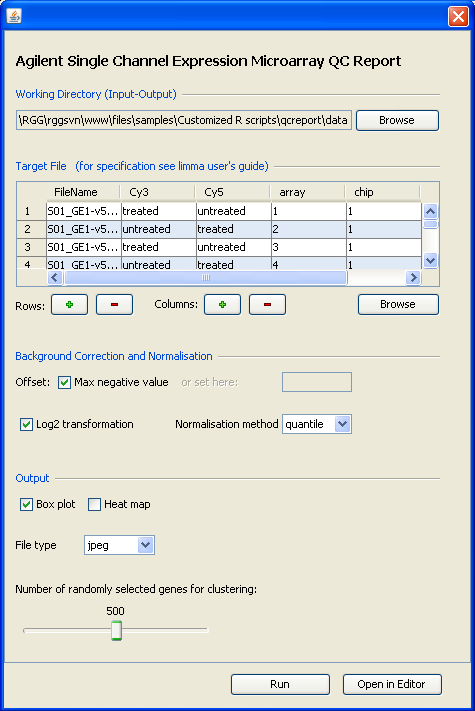### Latest news:

RGG has been published in BMC Bioinformatics. Read here

## Welcome to RGG!

`RGG is published in BMC Bioinformatics. Download and read the paper here`

RGG is a GUI generator for R scripts. RGG consists of an XML based GUI definition language and a Java based GUI engine. GUIs are generated in runtime from defined GUI tags that are embedded into the R script. User-GUI interaction is returned to the R code and replaces the XML-tags. RGG can be used with JGR (Java GUI for R) or as stand-alone software, and can be integrated to any software supporting R. The RGG project will further include the development of a web-based repository for R GUIs being developed by community.

RGG is available as standalone software (RGGRunner) and as an R package to be used with JGR. Here is a RGG-GUI and the corresponding R script:```setwd("C:/inputs")require(limma); require(gdata); require(pamr)targetfile = readTargets("Targets.txt")G = read.maimages(targetfile[,1],columns = list(G = "gMeanSignal", Gb = "gBGUsed",R = "gProcessedSignal", Rb ="gIsPosAndSignif")annotation= c("Row", "Col", "FeatureNum", "ProbeUID", "ControlType","ProbeName", "GeneName", "SystematicName"))Gbc <- backgroundCorrect(G, offset=(max(G\$Gb-G\$G)+1))norm <- normalizeBetweenArrays(log2(Gbc\$G), method='quantile')postscript('boxplots.ps')par(mfrow=c(1,2))boxplot(log2(G\$G)~col(G\$G), names=paste(G\$targets\$chip, G\$targets\$array,sep='_'), las=2, main='before Normalisation')boxplot(norm~col(norm), names=paste(G\$targets\$chip, G\$targets\$array, sep='_'),las=2, main='after Normalisation')dev.off()postscript('hm.ps')heatmap(norm[sample(1:nrow(norm), 5000), ], cexCol=0.5, labCol=as.character(G\$targets\$pat))dev.off()postscript('pc12.ps')plot(prcomp(t(norm))\$x[,1:2], col=as.numeric(G\$targets\$pat))dev.off()
```